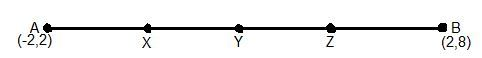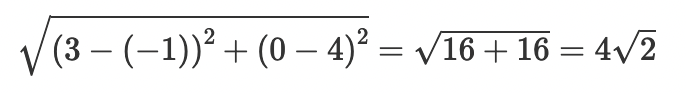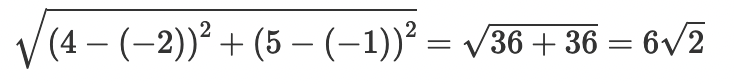# NCERT Class 10 Maths Chapter 7 – Coordinate Geometry Exercise 7.2 NCERT Solutions

## NCERT Class 10 Maths Chapter 7 – Coordinate Geometry Exercise 7.2 NCERT Solutions

The academic team at Neutronclasses has meticulously crafted NCERT solutions for Chapter 7 Exercise 7.2 of Class 10 Mathematics, which focuses on Coordinate Geometry. These solutions comprehensively cover all the exercises within this chapter. Presented below are the step-by-step solutions to all the questions found in the NCERT textbook for Chapter 7.

### Exercise 7.2

1. Find the coordinates of the point which divides the join of (- 1, 7) and (4, – 3) in the ratio 2:3.

Answer: Let P(x, y) be the required point. Using the section formula

Therefore the point is (1,3).

2. Find the coordinates of the points of trisection of the line segment joining (4, -1) and (-2, -3).

Let P (x1,y1) and Q (x2,y2) are the points of trisection of the line segment joining the given points i.e., AP = PQ = QB

Therefore, point P divides AB internally in the ratio 1:2.

Therefore P(x1,y1) = (2, -5/3)

Point Q divides AB internally in the ratio 2:1.

Q (x2 ,y2) = (0, -7/3)

3. To conduct Sports Day activities, in your rectangular shaped school ground ABCD, lines have been drawn with chalk powder at a distance of 1 m each. 100 flower pots have been placed at a distance of 1 m from each other along AD, as shown in the following figure. Niharika runs 1/4th the distance AD on the 2nd line and posts a green flag. Preet runs 1/5th the distance AD on the eighth line and posts a red flag. What is the distance between both the flags? If Rashmi has to post a blue flagexactly halfway between the line segment joining the two flags, where should she post her flag?

4. Find the ratio in which the line segment joining the points (-3, 10) and (6, – 8) is divided by (-1, 6).

Let the ratio in which the line segment joining ( -3, 10) and (6, -8) is divided by point ( -1, 6) be k:1.
Therefore, -1 = 6k-3/k+1
-k – 1 = 6k -3
7k = 2
k = 2/7
Therefore, the required ratio is 2:7.

5. Find the ratio in which the line segment joining A (1, – 5) and B (- 4, 5) is divided by the x-axis. Also find the coordinates of the point of division.

Let the ratio in which the line segment joining A (1, – 5) and B ( – 4, 5) is divided by x-axis be k:1.
Therefore, the coordinates of the point of division is (-4k+1/k+1, 5k-5/k+1).

We know that y-coordinate of any point on x-axis is 0.

∴ 5k-5/k+1 = 0

Therefore, x-axis divides it in the ratio 1:1.

To find the coordinates let’s substitute the value of k in equation(1)

Required point = [(- 4(1) + 1) / (1 + 1), (5(1) – 5) / (1 + 1)]

= [(- 4 + 1) / 2, (5 – 5) / 2]

= [- 3/2, 0]

6. If (1, 2), (4, y), (x, 6) and (3, 5) are the vertices of a parallelogram taken in order, find x and y.

Let A,B,C and D be the points (1,2) (4,y), (x,6) and (3,5) respectively.

7. Find the coordinates of a point A, where AB is the diameter of circle whose centre is (2, – 3) and B is (1, 4).

Let (x,y) be the coordinate of A.

Since AB is the diameter of the circle, the centre will be the mid-point of AB.

now, as centre is the mid-point of AB.

x-coordinate of centre = (2x+1)/2​

y-coordinate of centre = (2y+4)/2​

But given that centre of circle is (2,−3).

Therefore,

(2x+1)/2​=2⇒x=3

(2y+4​)/2=−3⇒y=−10

Thus the coordinate of A is (3,−10).

8. If A and B are (–2, –2) and (2, –4), respectively, find the coordinates of P such that AP = 3/7 AB and P lies on the line segment AB.

As given the coordinates of A(−2,−2) and B(2,−4) and P is a point lies on AB.

And AP = 3/7 ​AB

∴BP = 4/7

Then, ratio  of AP and PB = m1​:m2 ​= 3:4

Let the coordinates of P be (x,y).

∴ x = (m1​x2​ + m2​x1) / (m1​ + m2​)​​

⇒ x = (3 × 2 + 4 × (−2)) / (3 + 4) ​ = (6 − 8) / 7 ​= −2 / 7​

And y = (m1​y2​ + m2​y1) / (m1​ + m2​)​​​​

⇒ y = ((3 × (−4) + 4 × (−2)) / (3 + 4) ​= (−12−8) / 7 ​= −20 / 7​

∴ Coordinates of P = −2 / 7​, −20 / 7​

9. Find the coordinates of the points which divide the line segment joining A (- 2, 2) and B (2, 8) into four equal parts.From the figure, it can be observed that points X,Y,Z are dividing the line segment in a ratio 1:3,1:1,3:1 respectively.

Using Sectional Formula, we get,

Coordinates of X = ((1 × 2 + 3 × (−2)) / (1 + 3), (1 × 8 + 3 × 2) / (1 + 3))

= (−1, 7/2)

Coordinates of Y = (2 − 2) / 2, (2 + 8) / 2 = (0,5)

Coordinates of Z = ((3 × 2 + 1 × (−2)) / (1 + 3), (3 × 8 + 1 × 2) / (1 + 3)

= (1, 13/2)

10. Find the area of a rhombus if its vertices are (3, 0), (4, 5), (-1, 4) and (-2,-1) taken in order. [Hint: Area of a rhombus = 1/2(product of its diagonals)]